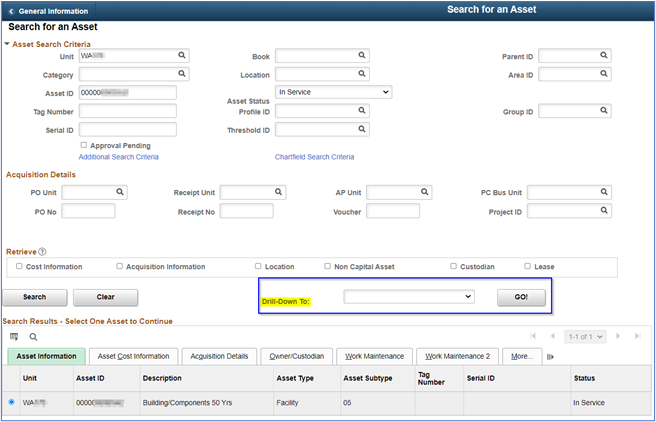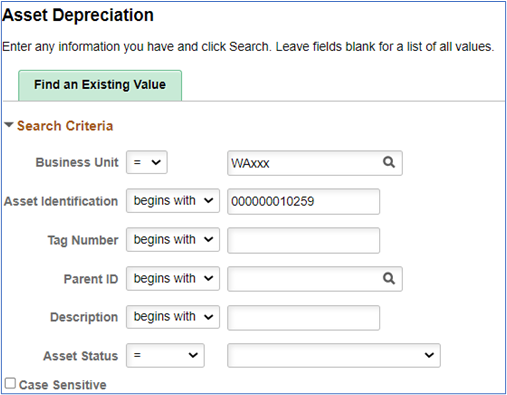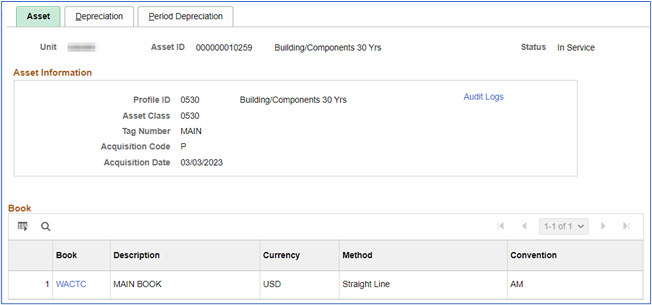# ctcLink Accounting Manual | 40.40.38 Depreciation Calculation

## 40.40.38 Depreciation Calculation

The complete the addition of an asset using Express Add, depreciation calculation must be run. This process must be run whether the asset is depreciable or not (e.g., land, non-depreciable collections).

Navigator >Asset Management > Depreciation > Processing> Calculate

If this is the first time you have run this process a new Run Control ID will need to be created.

It is not necessary to run this process after each single asset addition. If you have several assets to enter, it is permissible to enter all the assets and then run this process.

For step-by-step instructions see Processing Depreciation Calculations in the ctcLink Reference Center.

Upon completion of depreciation calculations, the asset will be viewable in Navigator >Asset Management > Search for an AssetThe “Drill-Down To:” feature allows the retrieval of the following information:

• Asset Accounting Entries
• Asset Basic Information
• Asset Cost History
• Asset Depreciation
• Asset Retirements
• Audit Logs Copy Asset
• Define Tax/Depr Criteria
• Print an Asset

Asset Depreciation information is available here using the Drill-Down feature or by using the Asset Depreciation screen found here: Navigator >Asset Management > Depreciation > Review Depreciation Info > Asset Depreciation.

Enter the Business Unit and Asset Identification or Tag Number or Description. If the complete Asset Identification, Tag Number, etc is entered, only that asset will be displayed.

If partial Asset Identification, Tag Number, etc. are entered the search will return a list of assets displaying the Basic Add screen.

The depreciation screens include Asset, Depreciation and Period Depreciation.

The Basic Add - Asset screen provides basic asset information:The Depreciation screen provides the following information: and the complete depreciation schedule (Yearly Depreciation).

### Basic Add - Depreciation Attributes### Basic Add - Net Book Value### Basic Add - Yearly Depreciation (Depreciation Schedule)

The table shows the depreciation for each year and the chartstring for recording depreciation. The accounts are inherited from the Accounting Entry Templates for the Asset and Cost Type (see Section 40.40.37 Enter Asset Cost Information above).### Basic Add - Period Depreciation

The Basic Add - Period Depreciation tab is used to view the anticipated monthly depreciation expense for the year selected.Page Manager: slocke@sbctc.edu